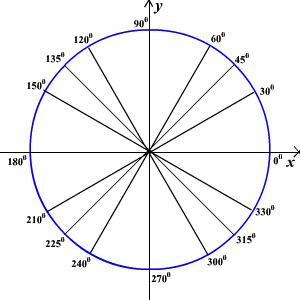# Degree Measure of an Angle

The measure of an angle is determined by the amount of rotation from the initial side to the terminal side.  One way to measure an angle is in terms of degrees .  A measure of one degree ( $1°$ ) is equivalent to a rotation of $\frac{1}{360}$ of a complete revolution.

To measure angles, it is convenient to mark degrees on the circumference of a circle .  Thus, a complete revolution is $360°$ , half a revolution is $180°$ , a quarter of a revolution is $90°$ and so forth.